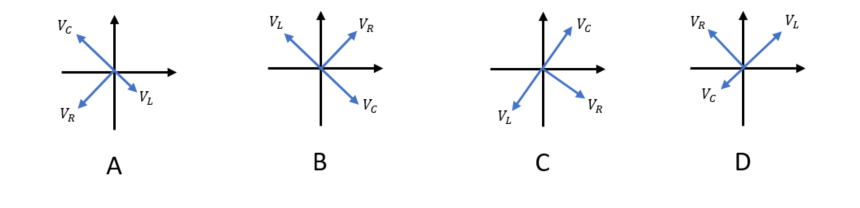# Problem: A series LRC circuit has a resistance of 15 Ω and an impedence of 20 Ω. Which of the following phasor diagrams could describe this circuit? Assume all phasors are rotating counter-clockwise.

91% (60 ratings)
###### Problem Details

A series LRC circuit has a resistance of 15 Ω and an impedence of 20 Ω. Which of the following phasor diagrams could describe this circuit? Assume all phasors are rotating counter-clockwise.Frequently Asked Questions

What scientific concept do you need to know in order to solve this problem?

Our tutors have indicated that to solve this problem you will need to apply the Phasors concept. You can view video lessons to learn Phasors. Or if you need more Phasors practice, you can also practice Phasors practice problems.

How long does this problem take to solve?

Our expert Physics tutor, Juan took 2 minutes and 39 seconds to solve this problem. You can follow their steps in the video explanation above.